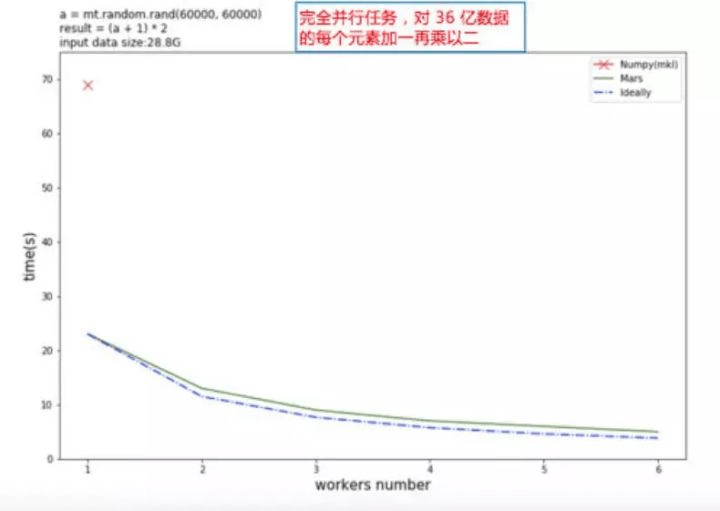# Alibaba Open-Sources Mars to Complement NumPy

Alibaba Cloud recently announced that it has open sourced Mars — its tensor-based framework for large-scale data computation — on Github. Mars can be regarded as “a parallel and distributed NumPy.” Mars can tile a large tensor into small chunks and describe the inner computation with a directed graph, enabling the running of parallel computation on a wide range of distributed environments, from a single machine to a cluster comprising thousands of machines.

“NumPy” is a beloved tool for the huge population of Python users who are mathematicians, engineers, etc. and working deeply in scientific computing. The NumPy Base N-dimensional array package usually contains:

• a powerful N-dimensional array object
• tools for integrating C/C++ and Fortran code
• useful linear algebra, Fourier transform, and random number capabilities

Alibaba Cloud recently announced that it has open sourced Mars — its tensor-based framework for large-scale data computation — on Github. Mars can be regarded as “a parallel and distributed NumPy.” Mars can tile a large tensor into small chunks and describe the inner computation with a directed graph, enabling the running of parallel computation on a wide range of distributed environments, from a single machine to a cluster comprising thousands of machines.

Alibaba Cloud Senior Engineer Xuye Qin introduced Mars from a performance perspective, boasting that “Mars can complete the computation on a 2.25T-size matrix and a 2.25T-size matrix multiplication in two hours.”

Mars’ key advantage is its ability to run matrix computation at a very large-scale, a forte that NumPy does not share. The chart below illustrates a simple experiment Alibaba developers ran to test Mars’ performance: They added one and then multiplied the result ((X+1)*2) for 3.6 billion data, then tested the change in computing time as the workers number (number of machines) increased. NumPy, represented by the red cross in the upper left, lags far behind Mars tensors, whose performance approaches ideal values.Mars currently supports a subset of NumPy interfaces, including:

• Arithmetic and mathematics: `+`, `-`, `*`, `/`, `exp`, `log`, etc.
• Reduction along axes (`sum`, `max`, `argmax`, etc).
• Most of the array creation routines (`empty`, `ones_like`, `diag`, etc). What’s more, Mars not only supports create array/tensor on GPU, but also supports create sparse tensor.
• Most of the array manipulation routines (`reshape`, `rollaxis`, `concatenate`, etc.)
• Basic indexing (indexing by ints, slices, newaxes, and Ellipsis)
• Fancy indexing along single axis with lists or numpy arrays, e.g. x[[1, 4, 8], :5]
• universal functions for elementwise operations.
• Linear algebra functions, including product (`dot`, `matmul`, etc.) and decomposition (`cholesky`, `svd`, etc.).

Mars tensor provides a familiar interface much like NumPy. Mars tensor can be installed with the code below:

`import mars.tensor as mta = mt.random.rand(1000, 2000)(a + 1).sum(axis=1).execute()`

Mars in distributed version is now available on Linux and Mac OS. Click this link for the Mars Github page.

Journalist: Tony Peng | Editor: Michael Sarazen